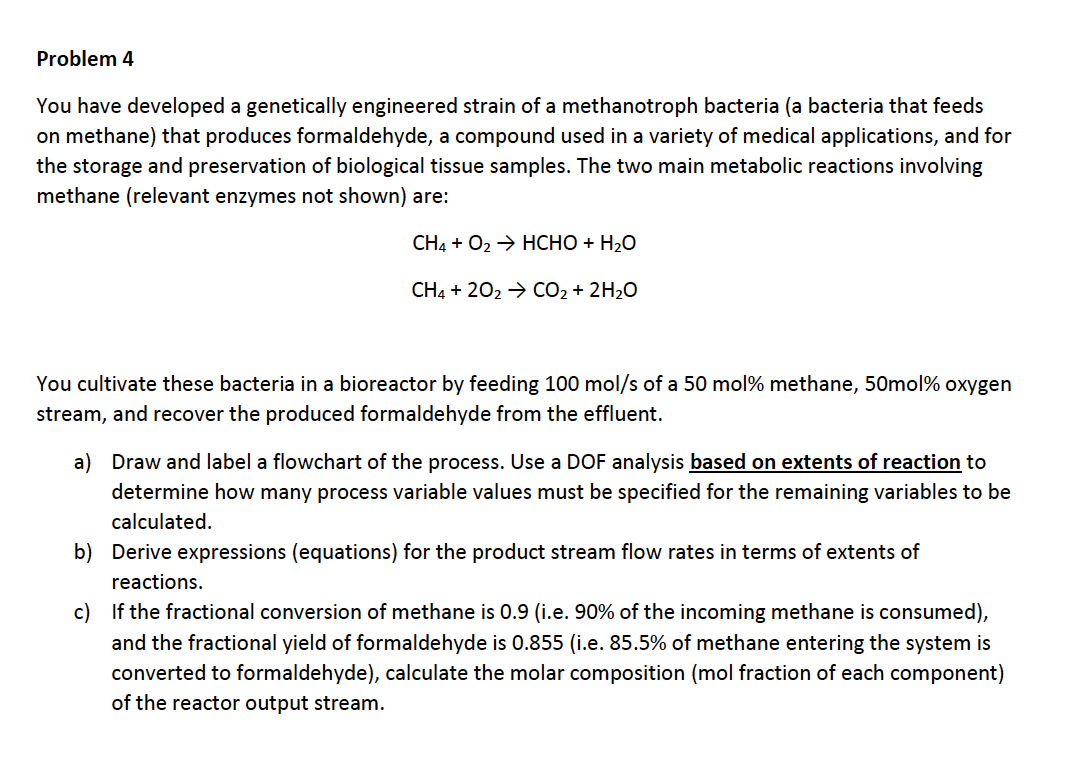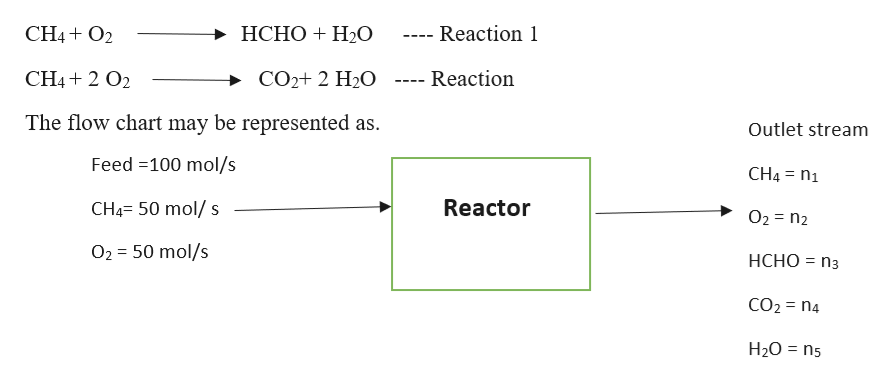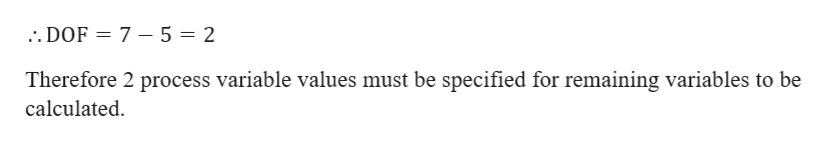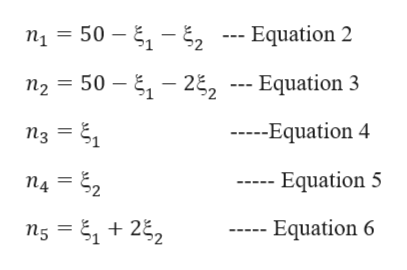# Problem 4You have developed a genetically engineered strain of a methanotroph bacteria (a bacteria that feedson methane) that produces formaldehyde, a compound used in a variety of medical applications, and forthe storage and preservation of biological tissue samples. The two main metabolic reactions involvingmethane (relevant enzymes not shown) are:CH4O2HCHO H20CH4 202 CO2 2H20You cultivate these bacteria in a bioreactor by feeding 100 mol/s of a 50 mol% methane, 50mol% oxygenstream, and recover the produced formaldehyde from the effluent.on extents of reaction toa) Draw and label a flowchart of the process. Use a DOF analysis badetermine how many process variable values must be specified for the remaining variables to becalculatedb) Derive expressions (equations) for the product stream flow rates in terms of extents ofreactionsc) If the fractional conversion of methane is 0.9 (i.e. 90% of the incoming methane is consumed),and the fractional yield of formaldehyde is 0.855 (i.e. 85.5% of methane entering the system isconverted to formaldehyde), calculate the molar composition (mol fraction of each component)of the reactor output stream.

Questionhelp_outlineImage TranscriptioncloseProblem 4 You have developed a genetically engineered strain of a methanotroph bacteria (a bacteria that feeds on methane) that produces formaldehyde, a compound used in a variety of medical applications, and for the storage and preservation of biological tissue samples. The two main metabolic reactions involving methane (relevant enzymes not shown) are: CH4O2HCHO H20 CH4 202 CO2 2H20 You cultivate these bacteria in a bioreactor by feeding 100 mol/s of a 50 mol% methane, 50mol% oxygen stream, and recover the produced formaldehyde from the effluent. on extents of reaction to a) Draw and label a flowchart of the process. Use a DOF analysis ba determine how many process variable values must be specified for the remaining variables to be calculated b) Derive expressions (equations) for the product stream flow rates in terms of extents of reactions c) If the fractional conversion of methane is 0.9 (i.e. 90% of the incoming methane is consumed), and the fractional yield of formaldehyde is 0.855 (i.e. 85.5% of methane entering the system is converted to formaldehyde), calculate the molar composition (mol fraction of each component) of the reactor output stream. fullscreen
check_circle

Step 1

Let n1 to n5 represent the molar flowrate of components in outlet stream.

The reactions taking place inside the reactor are as shown andlet x1 and x2 represent the extent of reaction for reaction 1 and 2 respectively.help_outlineImage TranscriptioncloseНСНО + Н0 CH4O2 Reaction 1 CH42 O2 Reaction CO2+ 2 H20 The flow chart may be represented as Outlet stream Feed 100 mol/s CH4 n1 CH4- 50 mol/ s Reactor O2 n2 O2 50 mol/s НСНО %3D Пз CO2 n4 H20 ns fullscreen
Step 2

Degree of freedom is given as in equation 1

DOF= (Number of unknowns) - (Total number of independent equations) – Equation 1

In the given problem there are 7 unknowns-  , n2, n3, n4, n5, x1, x2

The number of independent equations is equal to number of species in the system. Since there are 5 species the number of equations possible are 5.help_outlineImage Transcriptionclose.. DOF 7 - 5 = 2 Therefore 2 process variable values must be specified for remaining variables to be calculated fullscreen
Step 3

Part b

Product stream flow rates in terms of extent of reaction are given from equation 2 ...help_outlineImage Transcriptionclosen15 2- Equation 2 50 n2 50 -22 --- Equation 3 --Equation 4 n3 14 = 22 Equation 5 Equation 6 n5 22 fullscreen

### Want to see the full answer?

See Solution

#### Want to see this answer and more?

Solutions are written by subject experts who are available 24/7. Questions are typically answered within 1 hour.*

See Solution
*Response times may vary by subject and question.
Tagged in

### Process Calculations